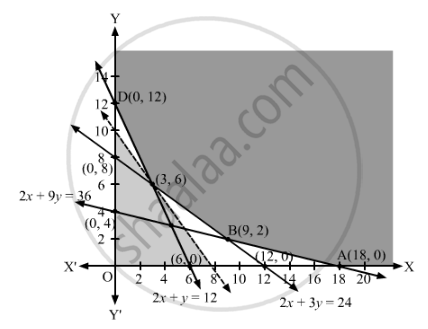# A Farmer Mixes Two Brands P and Q of Cattle Feed. Brand P, Costing ₹250 per Bag, Contains 2 Units of Nutritional Element A, 2.5 Units of Element B and 2 Units of Element C - Mathematics

Sum

A farmer mixes two brands P and Q of cattle feed. Brand P, costing ₹250 per bag, contains 2 units of nutritional element A, 2.5 units of element B and 2 units of element C. Brand Q costing ₹200 per bag contains 1.5 units of nutritional element A, 11.25 units of element B and 3 units of element C. The minimum requirements of nutrients A, B and C are 18 units, 45 units and 24 units respectively. Determine the number of bags of each brand which should be mixed in order to produce a mixture having a minimum cost per bag? What is the minimum cost of the mixture per bag?

#### Solution

Let x bags of brand P and bags of brand Q should be mixed to produce the mixture.

Each bag of brand P costs ₹250 and each bag of brand Q costs ₹200. Therefore, x bags of brand P and bags of brand Q costs ₹(250x + 200y).

Since each bag of brand P contains 3 units of nutritional element A and each bag of brand Q contains 1.5 units of nutritional element A, therefore, x bag of brand P and y bag of brand Q will contain (3x + 1.5y) units of nutritional element A. But, the minimum requirement of nutrients A is 18 units.

∴ 3x + 1.5≥ 18

⇒ 2x + ≥ 12

Similarly, x bag of brand P and y bag of brand Q will contain (2.5x + 11.25y) units of nutritional element B. But, the minimum requirement of nutrients B is 45 units.

∴ 2.5x + 11.25≥ 45

⇒ 2x + 9≥ 36

Also, x bag of brand P and y bag of brand Q will contain (2x + 3y) units of nutritional element B. But, the minimum requirement of nutrients C is 24 units.

∴ 2x + 3≥ 24

Thus, the given linear programming problem is

Minimise Z = 250x + 200y

subject to the constraints

2x + ≥ 12

2x + 9≥ 36

2x + 3≥ 24

x, y ≥ 0

The feasible region determined by the given constraints can be diagrammatically represented as,The coordinates of the corner points of the feasible region are A(18, 0), B(9, 2), C(3, 6) and D(0, 12).

The value of the objective function at these points are given in the following table.

 Corner Point Z = 250x + 200y (18, 0) 250 × 18 + 200 × 0 = 4500 (9, 2) 250 × 9 + 200 × 2 = 2650 (3, 6) 250 × 3 + 200 × 6 = 1950  → Minimum (0, 12) 250 × 0 + 200 × 12 = 2400

The smallest value of Z is 1950 which is obtained at (3, 6).

It can be seen that the open half-plane represented by 250x + 200y < 1950 or 5x + 4y < 39 has no common points with the feasible region.

So, 3 bags of brand P and 6 bags of brand Q should be used in the mixture to minimise the cost.

Hence, the minimum cost of the mixture per bag is ₹1950.

Concept: Graphical Method of Solving Linear Programming Problems
Is there an error in this question or solution?

#### APPEARS IN

RD Sharma Class 12 Maths
Chapter 30 Linear programming
Exercise 30.3 | Q 13 | Page 40